PHY294H - Lecture 14

Recommended problems: FGT 27.18, 27.32, 27.60

Electric current

Electric current describes the flow of charge. Thus we define current as charge per unit time, I = dQ/dt. When we write this formula we consider the net amount of charge that crosses a surface per unit time. Its units are C/s, however current is so important it has its own unit, the amp (A). The direction of positive current is the direction of flow of positive charge. This is the natural definition in terms of the electrostatics definitions that we have used. However it is a little unnatural in that in many cases it is the electrons which flow. Electron flow in a specified direction is negative current flow in that direction.

Current in terms of current density

Current density is the current per unit area. If all of the current density flows in the same direction, then the current is the current density times the area, ie. I=jAwhere A is the area. However if the current density has a variety of directions we have,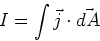(1)

where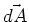is a small element of area and its direction is defined by the unit normal to the surface.

Current in terms of individual charges

Consider that we have a number density (number per unit volume), nq, of charged carriers which each have charge q. Suppose that these charge carriers have net drift'' velocity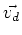in a specified direction. The current density is then given by,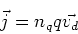(2)

Physical origin of drift velocity

The physical origin of the drift velocity is most easily seen using the so-called free electron model. In this model, metals are composed of a sea of free electrons which floats in between positive nuclei whose positions are essentially fixed (there are only some small vibrations about these fixed positions). The electrons move at high speed and in random directions. They scatter off each other, off the positive nuclei and from impurities. The time between scattering events isthe scattering time of the material. This varies from metal to metal. Now consider applying an electric field in a given direction. The electric field leads to an acceleration ma = - eE of each electron. Over a time, the velocity of the electron thus increases byin a direction opposite to the direction of the applied field. This is the drift velocity,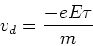(3)

Note that after each scattering event, the velocity of the electron is considered to be random. Using Eq. (2), we find another result which we shall refer to later,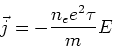(4)

Resistance, conductance

Ohm's, V=IR is famous, and was found experimentally. Using it we define the resistance R=V/I which has the unit the Ohm (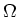). The resistor is a second circuit element which we need to learn how to analyse. Conductance is, in the simplest case, simply 1/R. We shall study predominantly linear resistors which obey Ohm's law. However many circuits use non-linear resistors and other non-linear circuit elements such as diodes and transistors.

Resistivity, conductivity

Resistivity is defined from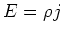. From this we see that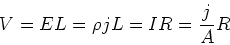(5)

The relation between resistance and resistivity is then,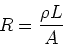(6)

In the simplest case the conductivity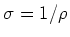.

Resistivity and conductivity are the intrinsic'' properties of a material. They do not depend on the geometry of a sample. In contrast, the resistance and conductance do depend on geometry (i.e. length and cross-sectional area). Material science and physics typically concentrate on designing atomic structure and morphology to control the resistivity and conductivity. Engineers typically engineer the geometry. However these days these area are merging in nano-engineering. Actually integrated circuits already combine engineering of the intrinsic properties (through silicon doping levels) as well as geometry.

Within the free electron model, using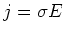, and Eq. (4), we have the elegant formula,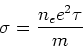(7)

Electric power

When a small amount of charge dq moves through a potential difference V, the change in potential energy is dU = dq V. In the case of resistors, this potential energy is dissipated as heat. The amount of power generated as heat is,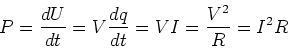(8)